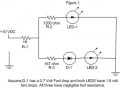# Current Calculation for Series Parallel circuit with diodes

#### mandacat

Joined Dec 1, 2016
2
Hi,
I couldn't find an explanation on the forum, so please forgive me if calculations for a circuit such as this were already explained.Simply ... ( or so I thought) - I want to find the current thru R-1.
If I eliminate D-1, I can follow the advise in the forum articles, using 8.2 volts (subtracting the 1.8 from the +10 VDC source) and calculate the resistance of R-1 and R-3 in parallel to find the total current thru R-1 ... BUT,

What is the method to use for this circuit and circuits like it, when the voltages are different across the parallel paths due to different type leds, batteries, etc., or similar devices with fixed voltages or voltage drops in those paths ?.

Thanks,
Bill

#### wayneh

Joined Sep 9, 2010
16,124
First we can assume that all diodes are conducting, and check it later.

Each diode will have a characteristic voltage drop. This is a function of current and temperature and isn't really known precisely. But for a problem like this we use standard values such as those given.

Then it comes down to voltages across resistors, and I think you probably know how to calculate that.

Finally, double check that with the calculated currents and voltages, there is enough voltage to start conduction in the diodes as assumed. For instance if the voltage at the junction of R1 and R2 is less than 1.8V, there cannot be any conduction through LED1. I doubt this will be the case but you need to look.

#### ian field

Joined Oct 27, 2012
6,539
Hi,
I couldn't find an explanation on the forum, so please forgive me if calculations for a circuit such as this were already explained.

View attachment 116230

Simply ... ( or so I thought) - I want to find the current thru R-1.
If I eliminate D-1, I can follow the advise in the forum articles, using 8.2 volts (subtracting the 1.8 from the +10 VDC source) and calculate the resistance of R-1 and R-3 in parallel to find the total current thru R-1 ... BUT,

What is the method to use for this circuit and circuits like it, when the voltages are different across the parallel paths due to different type leds, batteries, etc., or similar devices with fixed voltages or voltage drops in those paths ?.

Thanks,
Bill
You should probably leave out the 1k common to both paths - it just complicates the calculations for everything else. If safety is a concern; you can get very low rated fuses.

Each path has its own current limiting resistor, as such; each can be calculated in its own right.

If the series combination of diodes only accounts for half the supply voltage - you're dissipating as much energy as heat, as you are light.

Chaining more diodes to use up more voltage is less wasteful, but you make the current limiting "stiffer" - relatively speaking; a small change in supply voltage will cause a larger change in current. If the voltage is stabilised; that isn't a big problem. With stabilised supply, most of the problem is the temperature dependant Vf of the diodes.

#### mandacat

Joined Dec 1, 2016
2
Assuming the voltage drop of D1, LED1 and LED2 are known, and they are conducting. You can have the following relationship. Solve for V1, then from V1, you can get I1 = (10 - V1) / R1

View attachment 116231
Excellent !! Thanks so much for your quick reply and the solution. I plugged in the values and solved for V1 in the equation.
JFYI, this is an actual circuit from a rechargeable flashlight. I left out the NIMH battery and some other circuitry to simplify it, since the method was what I was looking for - and you aced it.

Thanks again,
Bill

•bug13